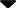Site Web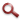You are here:    >

#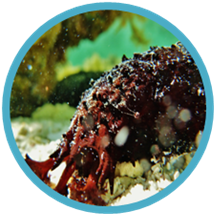Holothuria leucopsilota   (Brandt 1935)The Black Sea Cucumber Tanya Pernase (2011)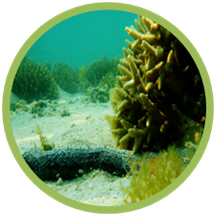## FEEDING

Introduction

Holothuria leucsopsilota is a non-select deposit feeder, ingesting the sandy substrate from the benthic habitat where it resides.  This species can often be seen slowly moving over large surface areas on the sea floor, ingesting the top most layer of sediment, playing a significant role in sediment turn-over, which is  important to the reef ecosystem (Ong Che 1990).  Specific studies on rates of consumption, carbon extraction and waste production are limited for the species.  However, Mangion et al. (2005) demonstrated sediment consumptions of 88.8 g dw.ind^-1.d^-1 and that the gut has a 10% efficiency at extracting organic matter from the sediment.

This study aims to increase understanding of feeding biology, by investigating total organic carbon content which H. lecusospilota is able to extract from the sediment. Furthermore, it will assist in understanding the role of this species in the transfer and recycling of organic matter and the carbon cycle.

Methods

Field sampling, aquarium experiments, dissections and sediment analysis took place on Heron Island Research Station at Heron Island (23°26’S, 151°55’E), the Great Barrier Reef, Australia.

Total organic carbon content extracted by H. leucospilota from the sediment

Individuals were collected from the same area of inner reef flat off Herron Island.  10 randomly chosen, fresh individuals were collected at 10am, during which time they were observed to be feeding for the three days respectively.  At this time, they were expected to have a near full gut, which was confirmed via a dissection.  The 10 cucumbers were split into 2 groups of 5 individuals and were kept for a 24 hour period in clean glass tanks (free from sediment and hence food), which had a constant input of sea water.  Faecal pallets were collected half an hour after the sea cucumbers were initially collected at 10:30am, then at three hours increments of 13:30, 16:30 and 1930.  Faecal pallets were dried in a dehydrator and the dry weight of each sample was recorded.  The samples were then cooked for a 9 hour period in a muffle furnace at 460 degrees Celsius. The weight was once again recorded and the difference in weight indicates the organic carbon content level.  The percentage extracted was then calculated.  This was repeated with fresh sea cucumbers from around the same area of reef flat for three consecutive days.

Organic carbon content of the sediment

Sediment was collected to compare the organic carbon content in the sand, to the content extracted by H. leucsospilota.  Sediment to a maximum depth of 10mm was collected from the site where the collected H. leucospilota were collected and the same methods of drying, cooking and weighing the sand were used.

Sediment sample from the intestine of H. leucospilota

An additional specimen of H. leucospilota was collected for dissection.  The intestine of the individual was extracted, which was at a filled level and the sand was removed from the membrane in what appeared to be three distinct sections.  These were the osphogogus, stomach and the third section was split into post stomach and anus.  Similarly to the other sediment collected it was dried, cooked and weighed.

Statistical analysis

Initially a Wilcoxon Signed-Rank Test was used to test whether there were any differences between samples collected from the two separate tanks.  This tested for consistency between efficiency of extraction of organic carbon content by H. leucospilota individuals.

An Independent Two-Sample T-test was then conducted to compare the carbon percentages between the sediment in the faecal pallets, reef flat sediment and intestine samples.   Initially there were a total of 12 averages of carbon content in the faecal samples.  However, there was one obvious outlier in the data, (with an average of 12.033% compared to the total mean of 3.051%)   which may have been a result of spilt sediment.  Therefore, this sample was removed from the results, accounting for 11 samples.  A preliminary test for the equality of variance was used to analyse whether the variance between groups was significantly different.

The percentages of carbon extracted were compared between all groups.

Faecal pallets verse reef flat sediment had values of F = 0.225, p = 0.022.  Therefore, a Two-Sample t-Test, assuming unequal variances was used.

Faecal pallets verse the intestine had values of F = 1.244, p = 0.480.  Therefore, a Two-Sample t-Test, assuming equal variances was used.

The intestine verse reef flat sediment had values of F = 4.870, p = 0.1098.  Therefore, a Two-Sample t-Test, assuming equal variances was used.

If H. leucospilota is efficient at extraction it is expected there should be a difference between intestine and faecal samples verse sediment levels.  Levels in the intestine and faecal pallets should be lower than in the sediment, with the faecal pallets having the lowest levels of all.

Image 1.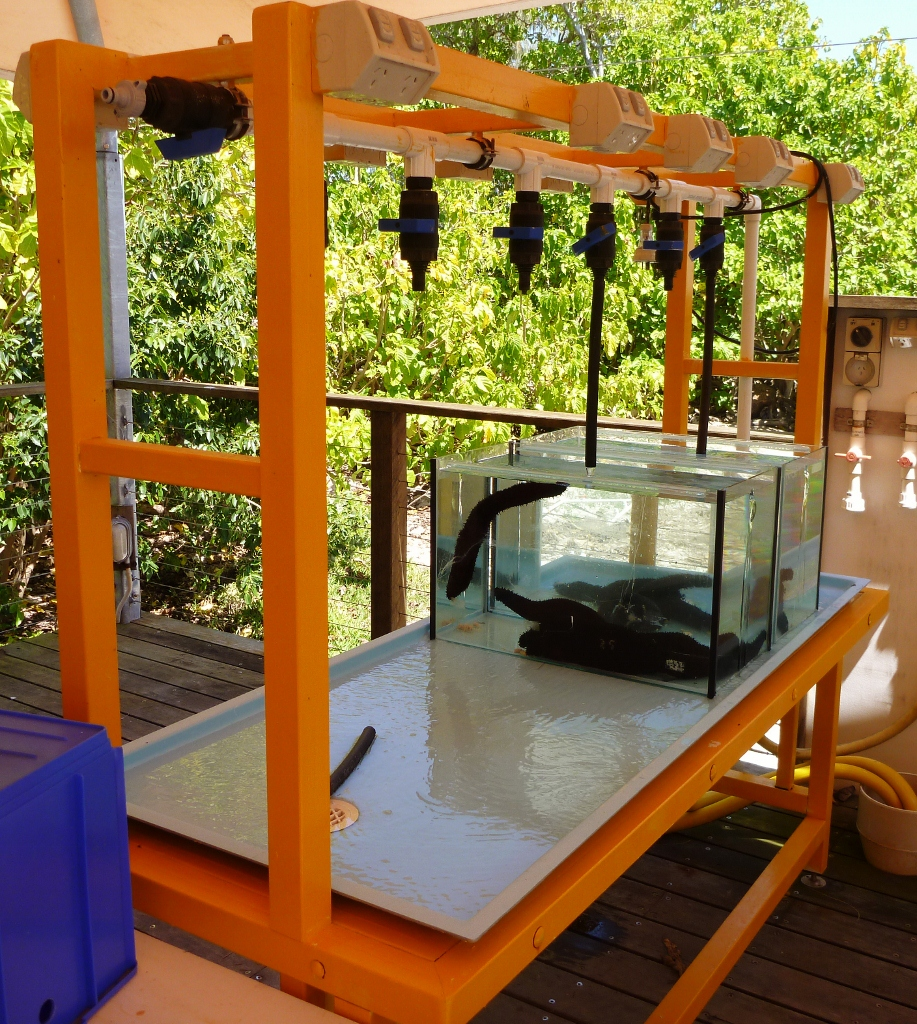Image 2.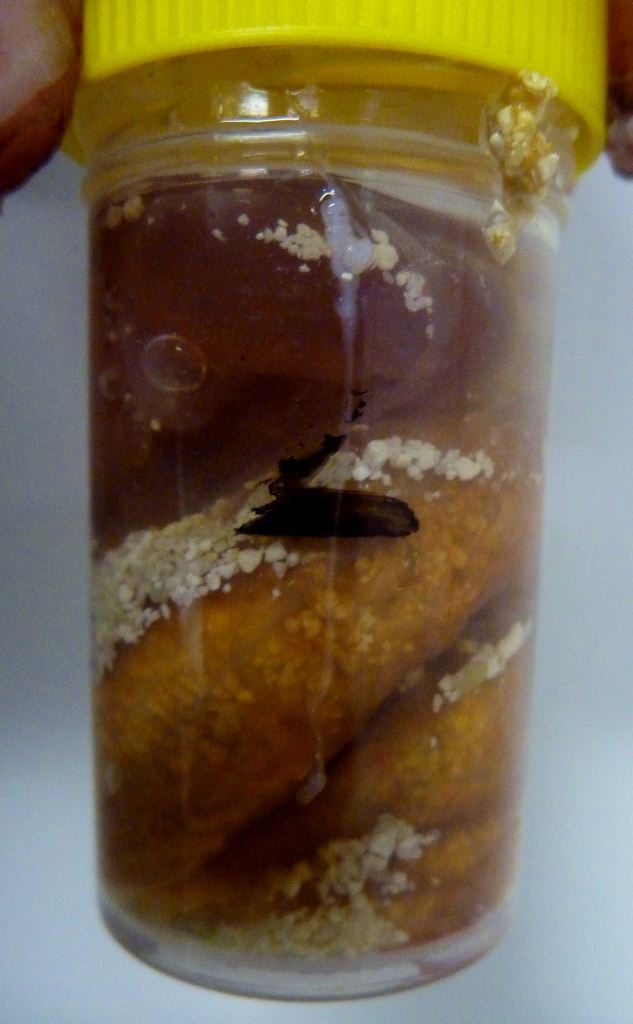Image 1. Sea cucumbers were set into two tanks, ech containing 10 individuals, at Heron Island Research Station.    Image 2.  Sample of intestine, looks similar to sediment collected.

Results

The Wilcoxon Signed-Rank Test confirmed the null hypothesis that there was no difference between the paired samples taken from the respective tanks over each time period.  The Test Statistics W was 28 and the sample number was 12.  As the Test Statistic W was greater than the W Critical, it can be confirmed that the collected H. leucospilota individauls had similar levels of consistency in regards to the amount of organic carbon content they extracted from the sediment.  Hence, averages of the two tanks were used from each time of day for further analysis of carbon extraction efficiency.

The difference between average carbon content in the faecal samples and sediment was only 0.938% and the difference between average carbon content in the intestine and sediment was only 0.286%.

Variable 1: Feacal samples
Variable 2. Sediment samples
Variable 3. Intestine sample

Table 1. Graph 1.  Comparisons of mean percentage of carbon in the difference samples.
The sediment was shown to have the highest carbon level, which was expected due still containing the whole carbon level.  Hence this also indicates the carbon level in the sediment, acting as a baseline to calculate extraction efficiency.

 Variable 1 Variable 2 Variable 3 Mean 3.051109 3.98913 3.70335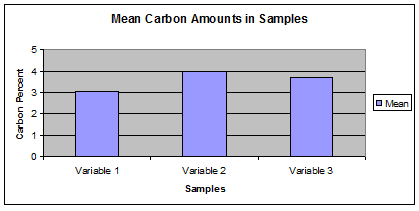Table 2. Graph 2. Overall assimilation efficiencies of the intestine and faecal/total extraction.
It is hypothesised the difference between efficiency is due to the intestine being in the process of extraction, where as the faecal samples show the overall assimilation levels.

 Faecal Sample 23.5144 Intestine 7.16397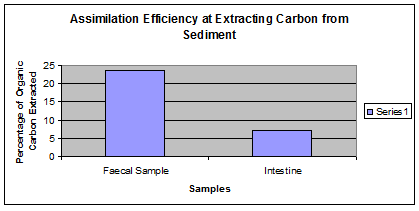Table 3.
 Sea Cucumber VS Sediment t-Test: Two-Sample Assuming Unequal Variances Variable 1 Variable 2 Mean 3.051109 3.98913 Variance 0.396164 1.551171 Observations 11 10 Hypothesized Mean Difference 0 df 13 t Stat -2.14559 P(T<=t) one-tail 0.025684 t Critical one-tail 1.770932 P(T<=t) two-tail 0.051369 t Critical two-tail 2.160368
IP value is less than 0.05, reject the null hypothesis of equal means.  Hence p values are just unequal.

Table 4.
 Sea Cucumber VS Intestine t-Test: Two-Sample Assuming Equal Variances Variable 1 Variable 3 Mean 3.051109 3.70335 Variance 0.396164 0.318499 Observations 11 4 Pooled Variance 0.378241 Hypothesized Mean Difference 0 df 13 t Stat -1.81637 P(T<=t) one-tail 0.046217 t Critical one-tail 1.770932 P(T<=t) two-tail 0.092434 t Critical two-tail 2.160368
 If p value is less than 0.05, reject the null hypothesis of equal means. Accept null hypothesis, indicating means are equal.

Table 5.
 Sediment VS Intestine t-Test: Two-Sample Assuming Unequal Variances Variable 2 Variable 3 Mean 3.98913 3.70335 Variance 1.551171 0.318499 Observations 10 4 Hypothesized Mean Difference 0 df 12 t Stat 0.589843 P(T<=t) one-tail 0.283118 t Critical one-tail 1.782287 P(T<=t) two-tail 0.566237 t Critical two-tail 2.178813
 If p value is less than 0.05, reject the null hypothesis of equal means. Therefore, this indicates the means are not equal.

Therefore overall it was found that statistically, feacal vs sediment samples were just unequal, faecal vs intestine were equal and sediment and intestine unequal.

Overall the sediment had the highest carbon level, which was to be expected, followed by the intestine and faecal samples, which also should be expected as it shows the progressive extraction of carbon from the sediment.

Holothuria leucospilota
was found to have an overall efficiency of 23.51% at extracting organic carbon from the sediment.

Conclusion

Analysis found that H. leucospilota had a 23.51% efficiency at extraction of organic carbon from the sediment.  The mean percentages of carbon in the faecal samples, sediment and intestine samples were 3.05%, 4% and 3.7% respectively. This is above levels found in other research (Mangion et al. 2005) .  Possible explainations may be levels of carbon in the sediment, making it easier for extraction or the ability of populations in the investigated area.  Hence further research is needed in understanding feeding of Holothuria leucospilota.